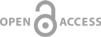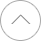10
MNiSW# THE SKETCH OF THE IDEA OF THE RESEARCH OF UNDERSTANDING AND USING THE LOGICAL STRUCTURE OF THE MATHEMATICAL STATEMENT.

 1 Instytut Matematyki, Uniwersytet Śląski
Studia Psychologiczne 2018;56(4):42–52
Publication date: 2018-04-03
Supplementary files
KEYWORDS
ABSTRACT
The process of teaching mathematics carries huge difficulties in understanding certain definitions, theorems or facts, which usually are written in adequate symbols. Proper understanding of information included in mathematical notation as well as logical structure of the notation itself is a basic rule of acquirement of mathematical knowledge. Wrong insight into the logical structure of mathematical sentences may be the cause of students failure during their learning and it can provide wrong impression on the studying case. The article introduces the sketch of the idea of the research about students’ understanding of the logical structure of mathematical problems and the way of using that structure in the process of correction false mathematical statements. Presented tests allow to distinguish preferences of the particular student – factual or logical aspects of mathematical truth. Places, where students make a correction in every sentence, tell us a lot about their choices – if they are interested only in factual meaning of the complex mathematical sentence or if they are also interested in logical structure of that sentence. Some results of the research and analysis of those results, as well as the main goal and short characterization of the research itself are presented in the following article.
Copy url
Share

 eISSN: 2300-8520 ISSN: 0081-685X